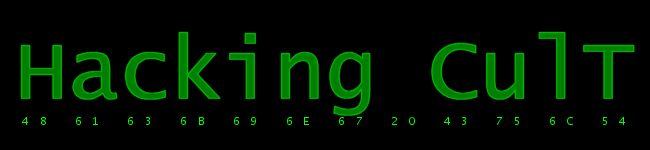Research / Megadrive Programming / Genesis security Control code Models >= 2 of Mega Drive / Genesis have a requirement of putting 'SEGA' to (\$A14000).l . Here's the code that will fix it (put it in the init section) ``` move.b (\$A10001).l,d0 ;version andi.b #\$F,d0 beq SkipSecurity ;skip if smd model = 1 move.l #'SEGA',(\$A14000).l SkipSecurity: ``` ChecksumChecksum is a word in ROM header that is calculated while compiling (in SEGA). While hacking, you probably encountered problems like red screen or 'WRONG CHECKSUM'. If you're asm hacking a rom, you have to find the checking routine and delete it. The routine is: ``` movea.l #\$200,a0 movea.l #\$7FFFF,a1 ; ROM End move.l (a1),d0 moveq #0,d1 loop: add.w (a0)+,d1 cmp.l a0,d0 bcc.s loop movea.l #\$18E,a1 ; Checksum cmp.w (a1),d1 bne.w WrongChecksum ```So to fix it, delete the 'bne WrongChecksum'. Depends on the rom, but usually the routine is exactly like that, because that's what's in the Mega Drive tech docs (by SEGA =P). But if you're hacking in a hex editor, you have to either calculate the checksum yourself or get a calculator. To calculate the checksum you do the following: 1. Start at \$200 2. Add a word to the checksum (ignore the upper bits) 3. Increment the offset 4. If offset < rom end, go to 2 5. Here's your checksum =)Back | Printer friendly<< 2. ROM header | 4. I/O ports >>